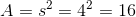# SSAT Elementary Level Math : How to find the area of a square

## Example Questions

### Example Question #11 : Quadrilaterals

Find the area of a square with a side of.Explanation:

Write the formula for area of a square.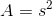Substitute the side length.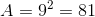### Example Question #12 : Quadrilaterals

What is the area of a square with the length of?Explanation:

Write the formula for area of a square.Plug in the side length.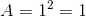### Example Question #13 : Quadrilaterals

Find the area of a square whose side length is 4.Explanation:

To solve for area, simply use the formula for finding area of a square.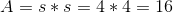### Example Question #14 : Quadrilaterals

Find the area of a square with side length 3.`Explanation:

To find the area of a rectangle, simply use the formula below: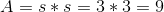### Example Question #15 : Quadrilaterals

Find the area of a square whose side length is 7.Explanation:

To solve, simply use the formula for the area of a square. Thus,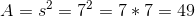where s=side length.

### Example Question #16 : Quadrilaterals

Find the area of a square whose side length is 0.1.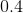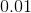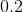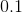Explanation:

To solve, simply use the formula for the area of a square below.

Thus,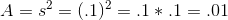### Example Question #17 : Quadrilaterals

Why is this shape a square?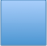Because it hasequal sides

Because its color is blue

Because it is big

Because it hasequal sides

Because it hasunequal sides

Because it hasequal sides

Explanation:

A square has 4 equal sides.

### Example Question #18 : Quadrilaterals

If a square has one side of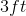, what is the area of the whole square?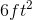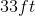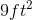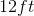Explanation:

To find the area of a square, you square the length of its sides or multiply it by itself.

Since the length is, the answer would be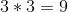.

Remember area always has square units.

### Example Question #11 : How To Find The Area Of A Square

Find the area of a square with side length 9.Explanation:

To solve, simply use the formula for the area of a square. Thus,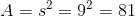As similarly stated in a prior problem in this set, if it helps you to envision smaller squares and adding them up for volume, thats perfectly ok! Some students also find success in knowing that area is two dimensions, and so you must multiply two variables together, in this case squaring the s.

### Example Question #20 : Quadrilaterals

Find the area of a square with side length 4.